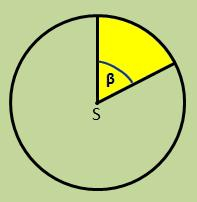# Circular 21273

The dragon has the shape of a circular section with a central angle of 40 ° and a radius of 35 cm. How much paper do we need to make if 10% is added to the curved ones?

S =  470.3662 cm2

### Step-by-step explanation:Did you find an error or inaccuracy? Feel free to write us. Thank you!

Tips for related online calculators
Need help calculating sum, simplifying, or multiplying fractions? Try our fraction calculator.
Our percentage calculator will help you quickly calculate various typical tasks with percentages.

#### Grade of the word problem:

We encourage you to watch this tutorial video on this math problem: